# Formula Calibration Method

## Calibrating with new nozzles

After installing the proper nozzle tips (See Steps 1-4 in Nozzle Tip Selection), you are ready to complete the calibration of the sprayer by following these additional steps:

### Step 5

Convert the GPM output for each nozzle (Step 3) to ounces per minute OPM:

GPM × 128 = OPM (1 gallon = 128 ounces)

For example: 0.25 GPM × 128 = 32 OPM

### Step 6

While the sprayer is stationary and the system pressurized, collect the output from the nozzle closest to the average output during the pre-calibration check (“quick check nozzle”) for 30 seconds and multiply by 2 or collect all of the output for one minute. Designating a quick check nozzle allows sprayer output to be measured quickly and easily any time the sprayer is used. However, the more nozzles you collect from, the more accurate the calibration. Calculate the average output from the nozzles sampled.

### Step 7

If the output measured  (Step 6) is within 5 percent of the desired OPM  (Step 5), the sprayer is calibrated accurately.  If changes are needed to bring the output within the 5 percent margin, you need to adjust the output (see Making Adjustments near the end of this section).

## Calibrating with existing nozzles:

### Step 1

Pressurize the sprayer to the desired operating pressure while it is parked.  Collect the output, in ounces, from a nozzle or several nozzles as described in Step 6 above to determine the nozzle flow rate in ounces per minute (OPM).

### Step 2

Convert OPM to GPM by dividing OPM by 128.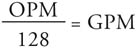### Step 3

Determine sprayer speed (MPH) as described in Nozzle Tip Selection.

### Step 4

Measure nozzle spacing (W) as described in Nozzle Tip Selection.

### Step 5

Calculate sprayer output in gallons per acre (GPA) by inserting the values determined in Steps 1-4 into the following formula: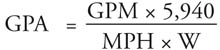To determine the equivalent gallons per 1,000 square feet, divide the GPA by 43.56 (the number of 1,000 sq. ft. units per acre; one acre = 43,560 sq. ft.).

A pesticide label specifies that the product be applied at a rate of 5 pints per acre in a spray volume of 30 gallons per acre. The average nozzle output from your boom sprayer is 56 OPM or 0.44 GPM (56/128). The sprayer speed is 4.5 MPH and nozzles are spaced 20 inches apart on the boom. What is the sprayer output in gallons per acre? How much pesticide should be mixed with water to treat 24 acres?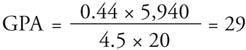To treat 24 acres at 29 gallons per acre, you will need a total spray solution of 696 gallons.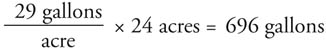At 5 pints per acre, the amount of pesticide needed is 120 pints.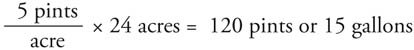For proper application, mix 15 gallons of pesticide with sufficient water to make 696 gallons of total spray solution.
Banding and directed crop foliar applications require a change in the value used for “W” in the formula.

## For Banding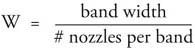### Banding Example

Banded herbicide application in 12-inch band over crop rows spaced 36 inches apart. There is one nozzle per band. The labeled (broadcast) rate is 5 pints per acre. The average nozzle output is 44 OPM or 0.34 GPM (44/128). The sprayer speed is 4.5 MPH. What is the sprayer output in gallons per acre? How much herbicide should be mixed with water to treat 24 acres in 12-inch bands?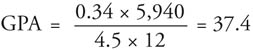This means the sprayer is applying 37.4 gallons per treated acre. When applying herbicides in a band, only a fraction of the total area of the field is treated. This area is referred to as treated acres. To determine how many treated acres there are in a specified field acreage, multiply the field acreage by the ratio of the band width to the row width: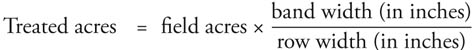In this example,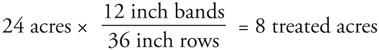Multiply the number of treated acres by the gallons per treated acre to determine the amount of total spray solution needed to treat the entire field: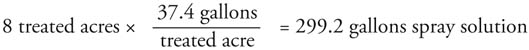Determine the amount of herbicide needed to treat the 30-acre field by multiplying the number of treated acres by the labeled broadcast rate: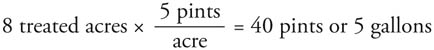Add 5 gallons of herbicide to 294.2 gallons of water (299.2 – 5) to treat the 24-acre field in 12-inch bands.
For directed crop foliar applications: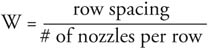### Foliar Crop Example

Directed insecticide application using 3 nozzles per row to a crop planted in 48-inch rows. The labeled (broadcast) rate is 2 quarts per acre. The average nozzle output is 36 OPM or 0.28 GPM (36/128). When checking the output on multiple-nozzle units, the GPM used in the formula is for each nozzle individually. The sprayer speed is 4.5 MPH. What is the sprayer output in gallons per acre? How much insecticide should be mixed with water to treat a 24 acre-field?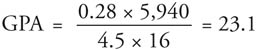To treat 24 acres at 23.1 gallons per acre, you will need a total spray solution of 554.4 gallons.

23.1 gallons per acre X 24 acres = 554.4 gallons

At 2 quarts per acre, the amount of insecticide needed is 48 quarts.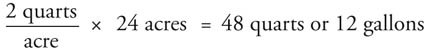For proper application, mix 12 gallons of insecticide with sufficient water to make 554.4 gallons of total spray solution.

If your sprayer’s output is not within 5 percent of the GPA on the label recommendation, then adjust the pressure, speed, or nozzle(s) according to the following guidelines:

• Adjust pressure if the measured output is between 5 percent and 10 percent of the recommended GPA. Reduce pressure to deliver less spray (lower GPA) or increase pressure to deliver more. Recheck output.
• Adjust travel speed if the measured output varies by more than 10 percent, but less than 25 percent. Slower speed delivers more spray, and faster speed delivers less. Measure speed under field conditions, insert new speed into the equation to determine GPA, and recheck output.
• If output varies by more than 25 percent, change nozzles and repeat the pre-calibration and calibration processes.

### Useful Formulas for Sprayer Adjustments

• GPA1 = measured application rate (gal/A)
• GPA2 = desired application rate (gal/A)
• MPH1 = measured travel speed (miles/hr)
• MPH2 = desired travel speed (miles/hr)
• psi1 = measured pressure (lb./sq.in.)
• psi2 = desired pressure (lb./sq.in.)

To determine the application rate (GPA) that will result from a change in nozzle pressure (psi):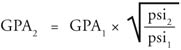(discharge rate (GPM) can be substituted for application rate (GPA) above)

To determine the desired pressure (psi) that will produce a desired application rate (GPA):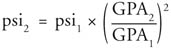(discharge rate (GPM) can be substituted for application rate (GPA) above)

To determine the application rate (GPA) that will result from a change in speed (MPH):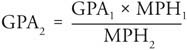To determine the desired speed (MPH) when changing application rate (GPA):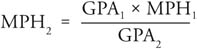Compiled by Wayne Buhler, PhD.